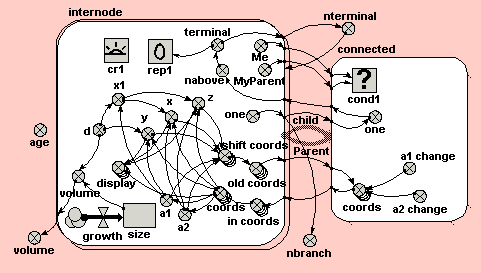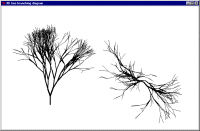# 3D tree branching model

ModelId:
branching1
SimileVersion:
5.9

This is a demonstration of Simile’s ability to handle fractal models.

L-system (Lindenmayer system) models are based on the idea that a complex structure can be obtained by repeatedly applying quite simple rules to a simple initial stucture. Thus, for example, the branching structure of a mature tree can be arrived at by applying simple rules to a seedling: rules such as "replace a bud by an extension to the branch, a side shoot, and two buds".

In the Simile implementation, we use a population submodel to hold the set of branch segments on the tree, and an association submodel to tell which segment is connectedto which other segment. As we iterate through time, each segment gives rise to two daughter segemnts, and thus the structure develops. Each segment is angled with respect to its parent in 3D: thus, the resulting structure is in 3D, and could be viewed as such in an appropriate 3D rendering environment.Equations:

Equations in ../Desktop

variable: age=time(1)-init_time(1)
variable: nbranch=sum({one}) where [{one}=internode/one]
variable: nterminal=sum({terminal}) where [{terminal}=internode/terminal]
variable: volume=sum({volume}) where [{volume}=internode/volume]

Equations in internode

variable: x1=d*sin(a1/57.3) where [a1=a1,d=d]
variable: y=element([coords],2)+d*cos(a1/57.3) where [[coords]=coords,d=d,a1=a1]
variable: x=element([coords],1)+x1*cos(a2/57.3) where [[coords]=coords,a2=a2,x1=x1]
variable: old coords=last([shift_coords]) where [[shift_coords]=shift coords]
variable: one=1
variable: in coords=[coords_0] where [{[coords]}=../connected/coords (to internode in Parent),[coords_0]=../connected/coords (to internode in child)]
variable: coords=(if index(1)== -1 then [0,0,0,0,0] else [in_coords]) where [[in_coords]=in coords]
if index(1)== -1 then [0,0,0,2] else [in_coords]
variable: nabove=sum({one}) where [{one}=../connected/one (to internode in Parent),one_0=../connected/one (to internode in child)]
variable: terminal=(if nabove==0 then 1 else 0) where [nabove=nabove]
variable: shift coords=[x,y,z,a1,a2] where [x=x,y=y,z=z,a1=a1,a2=a2]
variable: z=element([coords],3)+x1*sin(a2/57.3) where [[coords]=coords,a2=a2,x1=x1]
variable: a1=element([coords],4) where [[coords]=coords]
variable: a2=element([coords],5) where [[coords]=coords]
compartment: size=0.4
flow: growth=0.2
variable: d=rand_const(1.5,2)
variable: display=[element([coords],1),element([coords],2),element([coords],3),x,y,z] where [[coords]=coords,x=x,y=y,z=z]
variable: volume=d*3.14157*(size/2)^2 where [size=size,d=d]
creation: cr1=1
reproduction: rep1=(if terminal==1 then 2 else 0) where [terminal=terminal]
if terminal==1 and rand_var(0,1)<20/nterminal then 2 else 0
variable: MyParent=parent(1)
variable: Me=index(1)

Equations in connected

variable: coords=[old_coords]+[0,0,0,a1_change,a2_change] where [[old_coords]=../internode/old coords (from internode in Parent),[old_coords_0]=../internode/old coords (from internode in child),a1_change=a1 change,a2_change=a2 change]
variable: one=one_0 where [one=../internode/one (from internode in Parent),one_0=../internode/one (from internode in child)]
variable: a1 change=rand_const(-25,25)
variable: a2 change=rand_const(-40,40)

Results: